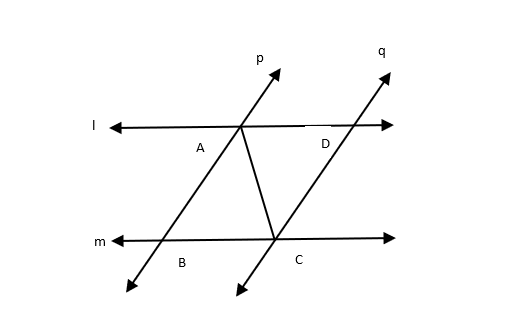Courses
Courses for Kids
Free study material
Free LIVE classes
MoreLIVE
Join Vedantu’s FREE Mastercalss

# l and m are two parallel lines intersected by another pair of parallel lines p and q. Show that $\Delta ABC \cong \Delta CDA$Verified
366k+ views
Hint- Use the properties of similarity of triangles.

Given: $l\parallel m$ and $p\parallel q$
Taking $l\parallel m$ and $AC$ is the traversal,
$\angle ACB = \angle CAD{\text{ }} \ldots \ldots \left( 1 \right){\text{ }}\left( {\because {\text{Alternate angles}}} \right)$
Considering $p\parallel q$ and $AC$ is the traversal,
$\angle BAC = \angle DCA{\text{ }} \ldots \ldots \left( 2 \right){\text{ }}\left( {\because {\text{Alternate angles}}} \right)$
In $\Delta ABC$ and $\Delta CDA$:
$\angle ACB = \angle CAD{\text{ }}\left( {{\text{from }}\left( 1 \right)} \right) \\ AC = CA {\text{ }}\left( {{\text{common}}} \right) \\ \angle BAC = \angle DCA{\text{ }}\left( {{\text{from }}\left( 2 \right)} \right) \\$
Therefore, using ASA congruence rule, we can say:
${\text{ }}\Delta ABC \cong \Delta CDA$
Hence Proved.

Note- When two triangles are congruent, they will have exactly the same three sides and exactly the same three angles. Although these equal sides and angles may not be at the exact same position. Also, there are criteria like SAS, ASA, SSS to prove triangles are congruent
Last updated date: 27th Sep 2023
Total views: 366k
Views today: 5.66k